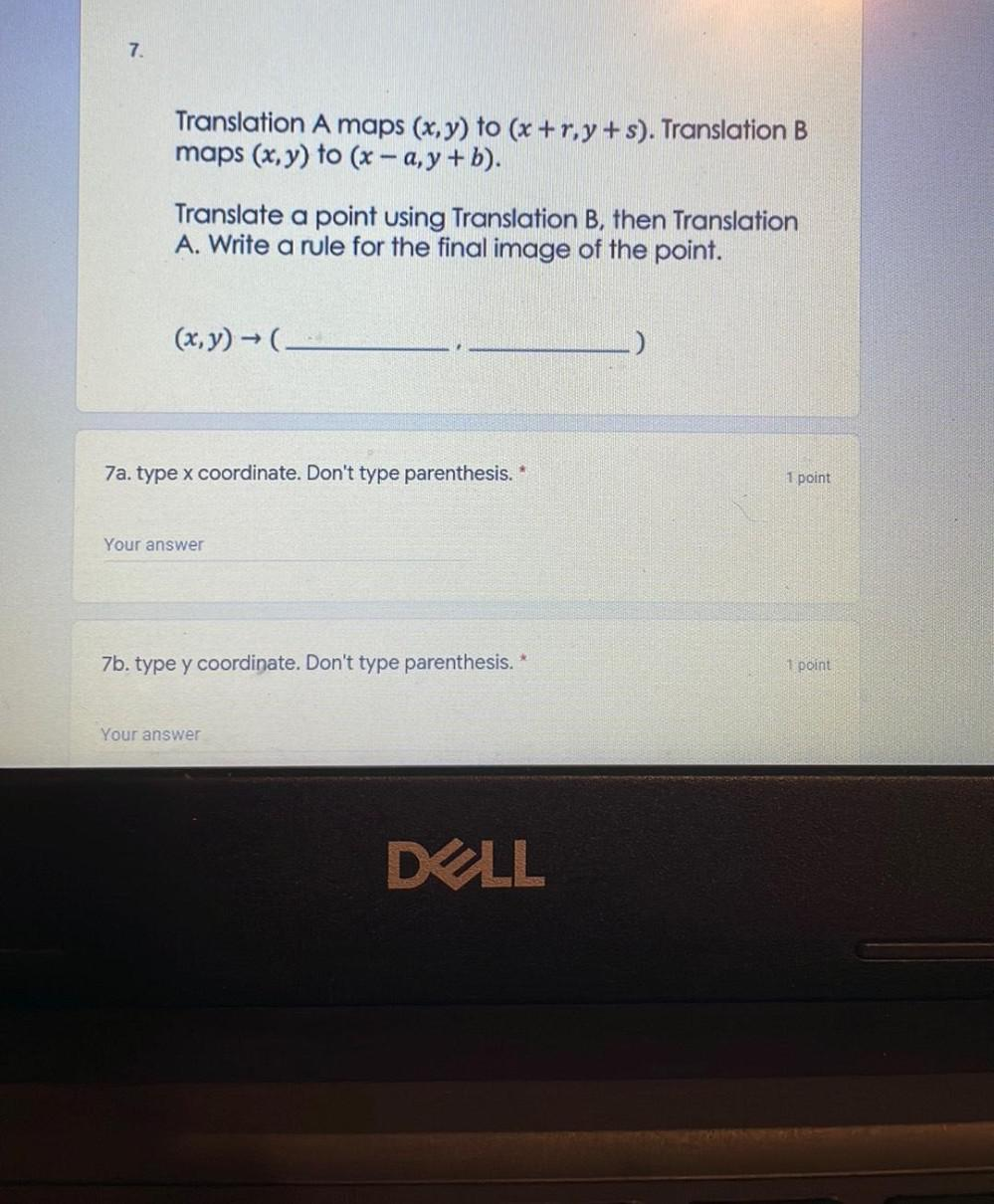Question:

# Translation A maps (x, y) to (x +r,y+s). Translation B maps (x, y) to (x – a,y+b). Translate a point using Translation B, then Translation A. Write a rule for the final image of the point. (x,y) → (.Translation A maps (x, y) to (x +r,y+s). Translation B maps (x, y) to (x – a,y+b). Translate a point using Translation B, then Translation A. Write a rule for the final image of the point. (x,y) → (._____________ ___________) a). type x coordinate. Don't type parenthesis. * b). type y coordinate. Don't type parenthesis.